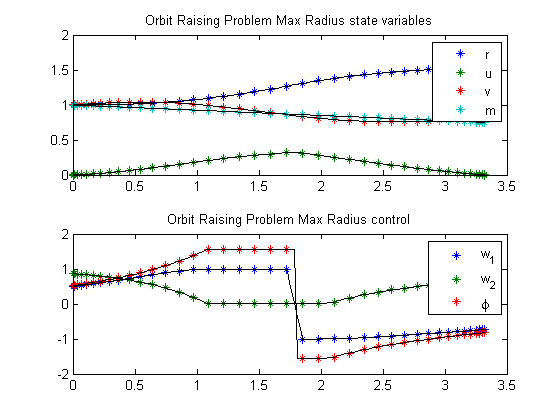78.1  Problem description

Maximize:

 J = r(tf)

subject to the dynamic constraints

 dr dt
= u
 du dt
=
 v2 r
 mmu r2
+T*
 w1 m

 dv dt
= −u*
 v r
+T*
 w2 m

 dm dt
= −
 T g0*ISP

the boundary conditions

 r(t0) = 1
 u(t0) = 1
 u(tf) = 0
v(t0) = (
 mmu r(t0)
)0.5
v(tf) = (
 mmu r(tf)
)0.5
 m(t0) = 1

and the path constraint

 w12+w22 = 1

The control pitch angel is not being used in this formulation. Instead two control variables (w1,w2) are used to for the thrust direction. A path constraint ensures that (w1,w2) is a unit vector.

Reference: 

78.2  Problem setup

t_F    = 3.32;
mmu    = 1;
m_0   = 1;   r_0     = 1;            u_0         = 0;
u_f   = 0;   v_0     = sqrt(mmu/r_0); rmin        = 0.9;
rmax  = 5;   umin    = -5;           umax        = 5;
vmin  = -5;  vmax    = 5;            mmax        = m_0;
mmin  = 0.1;

T  = 0.1405;
Ve = 1.8758;

toms t
p1 = tomPhase('p1', t, 0, t_F, 40);
setPhase(p1);

tomStates r u v m
tomControls w1 w2

% Initial guess
x0 = {icollocate({
r == r_0
u == u_0 + (u_f-u_0)*t/t_F
v == v_0
m == m_0})
collocate({w1 == 0; w2 == 1})};

% Boundary constraints
cbnd = {initial({
r == r_0
u == u_0
v == v_0
m == m_0
})
final({u == u_f
v - sqrt(mmu/r) == 0})};

% Box constraints
cbox = {
rmin <= icollocate(r) <= rmax
umin <= icollocate(u) <= umax
vmin <= icollocate(v) <= vmax
mmin <= icollocate(m) <= mmax
-1 <= collocate(w1) <= 1
-1 <= collocate(w2) <= 1};

% ODEs and path constraints
ceq = collocate({
dot(r) == u
dot(u) == v^2/r-mmu/r^2+T*w1/m
dot(v) == -u*v/r+T*w2/m
dot(m) == -T/Ve
w1^2+w2^2 == 1});

% Objective
objective = -final(r);

78.3  Solve the problem

options = struct;
options.name = 'Orbit Raising Problem Max Radius';
options.solver = 'snopt';
solution = ezsolve(objective, {cbox, cbnd, ceq}, x0, options);
Problem type appears to be: lpcon
Starting numeric solver
===== * * * =================================================================== * * *
TOMLAB - Tomlab Optimization Inc. Development license  999001. Valid to 2011-02-05
=====================================================================================
Problem: ---  1: Orbit Raising Problem Max Radius  f_k      -1.518744202740336600
sum(|constr|)      0.000017265841651215
f(x_k) + sum(|constr|)     -1.518726936898685300
f(x_0)     -0.999999999999991120

Solver: snopt.  EXIT=0.  INFORM=1.
SNOPT 7.2-5 NLP code
Optimality conditions satisfied

FuncEv    1 ConstrEv   59 ConJacEv   59 Iter   36 MinorIter  346
CPU time: 0.656250 sec. Elapsed time: 0.656000 sec.

78.4  Plot result

subplot(2,1,1)
ezplot([r u v m]);
legend('r','u','v','m');
title('Orbit Raising Problem Max Radius state variables');

subplot(2,1,2)
ezplot([w1 w2 atan2(w1,w2)])
legend('w_1', 'w_2', '\phi');
title('Orbit Raising Problem Max Radius control');